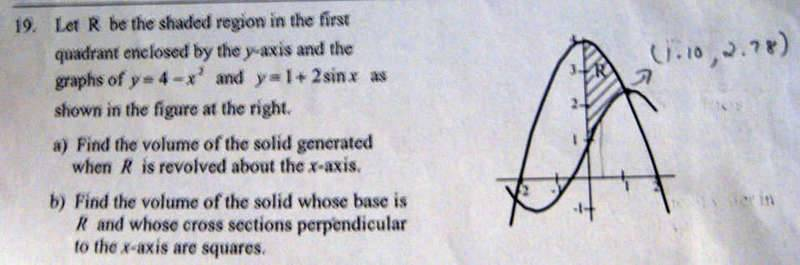# Another Integral Word Problem

• niravana21
In summary, the problem is trying to find the volume of a curve that intersects the y-axis at (1.10,2.78). TheAttempt at a Solution uses cross sections of the curve from 0 until it reaches 1.10, and then uses the area of a square to find the volume.f

## Homework Statement

Here is the problem:## The Attempt at a Solution

I found the intersection point to be (1.10,2.78). Now I don't know what to use for the lower limit.

We are revolving the curve around the x axis. Since the area of R is limited by the y-axis, this means our limits of integration will be the x-coordinate where the two graphs intersect the y-axis and the point where they intersect each other.

What method of finding volume are you using? Cross sections or cylindrical shell? If using cross sections this will be a dx problem; if using the shell method this will be a dy problem. For this problem I would use the cross section/disk method and thus use x-coordinates as limits of integration. For a, this will be a washer.

Ya the upper limit is the point of intersection but what do you mean by when the graphs interse t the y axis? You can't have 3 limits?

Edit: so from 0 to 1.10?

It is zero--since we are bounded by the y-axis and the intersection, our limits of integration are 0,1.10.

When we find the volume we are going to add cross sections of the curve from 0 until we reach 1.10. Think of a layer cake--the bottom is zero and the top is the intersection of the curve. Turn it sideways and let the axis down the middle be the x-axis, and that is what we are doing.

Last edited:
And what about the second part?

We'll let f(x)=4-x2 and g(x)=1+2sin(x)
Use the same limits of integration, but a different cross section. The area of a square is A=s2. In this case s=f(x)-g(x), f(x)>g(x) on the interval of the limits of integration. Take Sab[f(x)-g(x)]2dx.

For a, remember to treat it as a washer. V=pi*Sab[f(x)]2-[g(x)]2dx.

just a general question, for both parts if I use the limits 0 to 1.10, won't that also include the area under the curve for 1+2sin(x) curve until x=1.10?

If you treat it as a washer for a, you will have subtracted the volume of 1+2sin(x). For part b, you also subtract the area of 1+2sin(x). 4-x2 includes the area/volume resulting from 1+2sin(x), so by subtracting it we get the area of R.

Earlier I accidently used 2.78 instead of 1.10...fixed my above post.